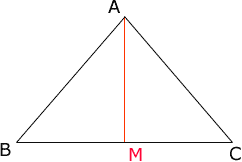Quandaries and Queries I have a triangle that with a base of 60’ and the two sides of 37’. I know the formula for area is A=½ (b*h) but how do I find the height?? Hi Martha, Since this is an isosceles triangle you can find the height using Pythagoras Theorem. First find the midpoint M of the 60' base and then join this point to the vertex A of the triangle as in the diagram below.Since |AB| = |CA| , |BM| = |MC|, and |AM| = |AM|, the triangles ABM and AMC are congruent. Hence angles BMA and AMC are equal. Since there sum is 180o each is 90o and thus triangle ABM is a right triangle. Hence Pythagoras Theorem tells us that |AB|2 = |BM|2 + |MA|2 that is 372 = 30 2 + |MA|2 and hence the height |MA| of the triangle is |MA| = Sqrt(372 - 302) = 21,66 square feet. The fact that the triangle is isosceles was needed here to show that triangle ABM is a right triangle. An alternate procedure to finding the area is to use Heron's Formula. Penny Go to Math Central